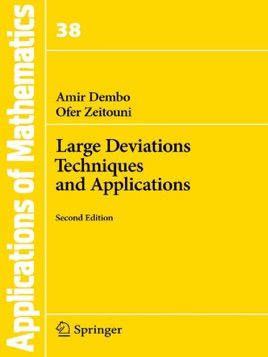• 32,99 €

## Description de l’éditeur

The theory of large deviations deals with the evaluation, for a family of probability measures parameterized by a real valued variable, of the probabilities of events which decay exponentially in the parameter. Originally developed in the context of statistical mechanics and of (random) dynamical systems, it proved to be a powerful tool in the analysis of systems where the combined effects of random perturbations lead to a behavior significantly different from the noiseless case. The volume complements the central elements of this theory with selected applications in communication and control systems, bio-molecular sequence analysis, hypothesis testing problems in statistics, and the Gibbs conditioning principle in statistical mechanics.

Starting with the definition of the large deviation principle (LDP), the authors provide an overview of large deviation theorems in ${{\rm I\!R}}^d$ followed by their application. In a more abstract setup where the underlying variables take values in a topological space, the authors provide a collection of methods aimed at establishing the LDP, such as transformations of the LDP, relations between the LDP and Laplace's method for the evaluation for exponential integrals, properties of the LDP in topological vector spaces, and the behavior of the LDP under projective limits. They then turn to the study of the LDP for the sample paths of certain stochastic processes and the application of such LDP's to the problem of the exit of randomly perturbed solutions of differential equations from the domain of attraction of stable equilibria. They conclude with the LDP for the empirical measure of (discrete time) random processes: Sanov's theorem for the empirical measure of an i.i.d. sample, its extensions to Markov processes and mixing sequences and their application.

The present soft cover edition is a corrected printing of the 1998 edition.

Amir Dembo is a Professor of Mathematics and of Statistics at Stanford University. Ofer Zeitouni is a Professor of Mathematics at the Weizmann Institute of Science and at the University of Minnesota.